# Cumulative frequency analysis

﻿
Cumulative frequency analysis

Cumulative frequency analysis is the applcation of estimation theory to exceedance probability (or equivalently to its complement). The complement, the non-exceedance probability concerns the frequency of occurrence of values of a phenomenon staying below a reference value. The phenomenon may be time or space dependent. Cumulative frequency is also called frequency of non−exceedance.

Cumulative frequency analysis is done to obtain insight into how often a certain phenomenon (feature) is below a certain value. This may help in describing or explaining a situation in which the phenomenon is involved, or in planning interventions, for example in flood protection.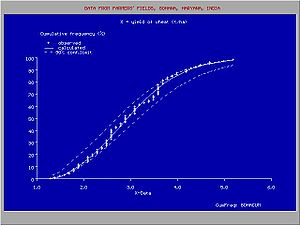Graphic illustration of cumulative frequency distribution, adapted cumulative probability distribution, and confidence intervals

## Principles

### Definition

Frequency analysis  applies to a record of observed data on a variable phenomenon. The record may be time dependent (e.g. rainfall measured in one spot) or space dependent (e.g. crop yields in an area) or otherwise.

The variable phenomenon is modelled by a random variable X and the parameter pC of interest is the non-exceeding probability:$p_C=P(X\le X_r),$

in which Xr is a given reference value.

The record of observed data consists of a random sample$X_1, X_2, \dots, X_N$ taken from X.

In this context the number of times the reference value Xr is not exceeded in the sample is called the cumulative frequency MXr. The relative cumulative frequency is this number divided by the sample size N and is sometimes denoted as Fc(XXr), or briefly as Fc(Xr). It is calculatd as;$F_C(X_r) = \frac{M_{X_r}}N.$

Briefly the expression can be noted as: FC = M / N.

When Xr=Xmax, where Xmax is the maximum value observed, it is found that Fc=1, because M=N.

### Estimation

The parameter pC is estimated on the basis of the relative cumulative frequency Fc. The estimate is:$\frac{M_{X_r}}{N+1}=\frac N{N+1}F_C(X_r)=$

In brief:

• Fc = M / (N+1)

The denominator N+1 is introduced instead of N to create the possibility for X to be greater than Xmax as now Pc(Xmax) is less than 1. There exist also other proposals for the denominator (see plotting positions), but they are claimed to be incorrect.

## Fitting of probability distributions

### Continuous distributions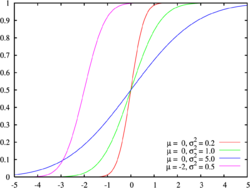Different cumulative normal probability distributions with their parameters

To present the cumulative frequency distribution as a continuous mathematical equation instead of a discrete set of data, one may try to fit the cumulative frequency distribution to a known cumulative probability distribution,.
If successful, the known equation is enough to report the frequency distribution and a table of data will not be required. Further, the equation helps interpolation and extrapolation.However, care should be taken with extrapolating a cumulative frequency distribution, because this may be a source of errors. One possible error is that the frequency distribution does not follow the selected probability distribution any more beyond the range of the observed data.

Any equation that gives the value 1 when integrated from a lower limit to an upper limit agreeing well with the data range, can be used as a probability distribution for fitting. A sample of probability distributions that may be used can be found in probability distributions.

Probability distributions can be fitted by several methods, for example:

• the parametric method, determining the parameters like mean and standard deviation from the X data using the method of moments, the maximum likelihood method and the method of probability weighted moments.
• the regression method, linearizing the probability distribution through transformation and determining the parameters from a linear regression of the transformed Pc (obtained from ranking) on the transformed X data.

Application of both types of methods using for example the

often shows that a number of distributions fit the data well and do not yield significantly different results, while the differences between them may be small compared to the width of the confidence interval. This illustrates that it may be difficult to determine which distribution gives better results.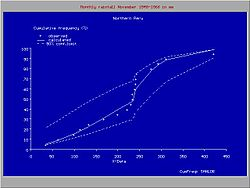Cumulative frequency distribution with a discontinuity

### Discontinuous distributions

Sometimes it is possible to fit one type of probability distribution to the lower part of the data range and another type to the higher part, separated by a breakpoint, whereby the overall fit is improved.

The figure gives an example of a useful introduction of a such a discontinuous distribution for rainfall data in northern Peru, where the climate is subject to the behavior Pacific ocean current El Niño. When the Niño extends to the south of Ecuador and enters the ocean along the coast of Peru, the climate in Northern Peru becomes tropical and wet. When the Niño does not reach Peru, the climate is semi−arid. For this reason, the higher rainfalls follow a different frequency distribution than the lower rainfalls.

## Prediction

### Uncertainty

When a cumulative frequency distribution is derived from a record of data, it can be questioned if it can be used for predictions. For example, given a distribution of river discharges for the years 1950 to 2000, can this distribution be used to predict how often a certain river discharge will be exceeded in the years 2000 to 2050? The answer is yes, provided that the environmental conditions do not change. If the environmental conditions do change, such as alterations in the infrastructure of the river's watershed or in the rainfall pattern due to climatic changes, the prediction on the basis of the historical record is subject to a systematic error. Even when there is no systematic error, there may be a random error, because by chance the observed discharges during 1950 − 2000 may have been higher or lower than normal, while on the other hand the discharges from 2000 to 2050 may by chance be lower or higher than normal.

### Confidence intervals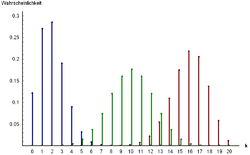Binomial distributions for Pc=0.1 (blue), 0.5 (green) and 0.8 (red) in a sample of size N=20. The distribution is symmetrical only when Pc = 0.5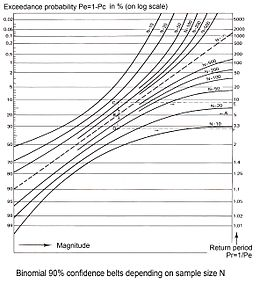90% Binomial confidence belts on a log scale.

Probability theory can help to estimate the range in which the random error may be. In the case of cumulative frequency there are only two possibilities: a certain reference value X is exceeded or it is not exceeded. The sum of exceedance frequency and cumulative frequency is 1 or 100%. Therefore the binomial distribution can be used in estimating the range of the random error.

According to the normal theory, the binomial distribution can be approximated and for large N standard deviation Sd can be calculated as follows:

• Sd =√{Pc(1−Pc)/N}

where Pc is the cumulative probability and N is the number of data. It is seen that the standard deviation Sd reduces at an increasing number of observations N.

The determination of the confidence interval of Pc makes use of Student's t-test (t). The value of t depends on the number of data and the confidence level of the estimate of the confidence interval. Then, the lower (L) and upper (U) confidence limits of Pc in a symmetrical distribution are found from:

• L = Pc − t.Sd.
• U = Pc + t.Sd

This is known as Wald interval . However, the binomial distribution is only symmetrical around the mean when Pc = 0.5, but it becomes asymmetrical and more and more skew when Pc approaches 0 or 1. Therefore, by approximation, Pc and 1−Pc can be used as weight factors in the assignation of t.Sd to L and U :

• L  = Pc − 2Pc.t.Sd
• U = Pc + 2(1−Pc).t.Sd

where it can be seen that these expressions for Pc = 0.5 are the same as the previous ones.

 Example N = 25, Pc = 0.8, Sd = 0.08, confidence level is 90%, t = 1.71, L = 0.70, U = 0.85 Thus, with 90% confidence, it is found that 0.70 < Pc < 0.85 Still, there is 10% chance that Pc < 0.70, or Pc > 0.85

Wald interval is known to perform poorly  .

Wilson score interval provides confidence interval for binomial distributions based on score tests and has better sample coverage, see  and binomial proportion confidence interval for a more detailed overview.

### Return period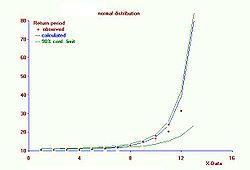Return periods and confidence belt. The curve of the return periods increases exponentially.

The cumulative probability Pc can also be called probability of non−exceedance. The probability of exceedance Pe is found from:

• Pe = 1 − Pc.

The return period T defined as:

• T = 1/Pe

and indicates the expected number of observations that have to be done again to find the value of the variable in study greater than the value used for T.
The upper (TU) and lower (TL) confidence limits of return periods can be found respectively as:

• TU = 1/(1−U)
• TL = 1/(1−L)

For extreme values of the variable in study, U is close to 1 and small changes in U originate large changes in TU. Hence, the estimated return period of extreme values is subject to a large random error. Moreover, the confidence intervals found hold for a long term prediction. For predictions at a shorter run, the confidence intervals UL and TUTL may actually be wider. Together with the limited certainty (less than 100%) used in the t−test, this explains why, for example, a 100−year rainfall might occur twice in 10 years.Nine return period curves of 50-year samples from a theoretical 1000 year record (base line)

The strict notion of return period actually has a meaning only when it concerns a time−dependent phenomenon, like point rainfall. The return period then corresponds to the expected waiting time until the exceedance occurs again. The return period has the same dimension as the length of time for which each observation is representative. For example, when the observations concern daily rainfalls, the return period is expressed in days, and for yearly rainfalls it is in years.

### Need of confidence belts

The figure shows the variation that may occur when obtaining samples of a variate that follows a certain probability distribution. The data were provided by Benson .

The confidence belt around an experimental cumulative frequency or return period curve gives an impression of the region in which the true distribution may be found.

Also, it clarifies that the experimentally found best fitting probability distribution may deviate from the true distribution.

## Histogram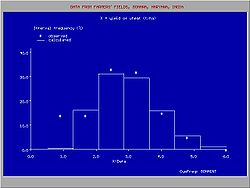Histogram derived from the adapted cumulative probability distribution

The observed data can be arranged in classes or groups with serial number k. Each group has a lower limit (Lk) and an upper limit (Uk). When the class (k) contains mk data and the total number of data is N, then the relative class or group frequency is found from:

• Fg(Lk<XUk)  = mk / N

or briefly:

• Fgk = m/N

or in percentage:

• Fg(%) = 100m/N

The presentation of all class frequencies gives a frequency distribution or histogram. Histograms, even when made from the same record, are different for different class limits.

The histogram can also be derived from the fitted cumulative probability distribution:

• Pgk = Pc(Uk) − Pc(Lk)

There may be a difference between Fgk and Pgk due to the deviations of the observed data from the fitted distribution (see figure).

Wikimedia Foundation. 2010.

### Look at other dictionaries:

• Cumulative frequency — may refer to: Cumulative distribution function Cumulative frequency analysis This disambiguation page lists articles associated with the same title. If an internal link led you here, you may …   Wikipedia

• Cumulative distribution function — for the normal distributions in the image below …   Wikipedia

• Frequency — For other uses, see Frequency (disambiguation). Three cyclically flashing lights, from lowest frequency (top) to highest frequency (bottom). f is the frequency in hertz (Hz), meaning the number of cycles per second. T is the period in seconds (s) …   Wikipedia

• Frequency distribution — In statistics, a frequency distribution is an arrangement of the values that one or more variables take in a sample. Each entry in the table contains the frequency or count of the occurrences of values within a particular group or interval, and… …   Wikipedia

• Cumulative incidence — or incidence proportion is a measure of frequency, as in epidemiology, where it is a measure of disease frequency during a period of time. Where the period of time considered is an entire lifetime, the incidence proportion is called lifetime risk …   Wikipedia

• Survival analysis — is a branch of statistics which deals with death in biological organisms and failure in mechanical systems. This topic is called reliability theory or reliability analysis in engineering, and duration analysis or duration modeling in economics or …   Wikipedia

• Principal component analysis — PCA of a multivariate Gaussian distribution centered at (1,3) with a standard deviation of 3 in roughly the (0.878, 0.478) direction and of 1 in the orthogonal direction. The vectors shown are the eigenvectors of the covariance matrix scaled by… …   Wikipedia

• Medical analysis of circumcision — Numerous medical studies have examined the effects of male circumcision with mixed opinions regarding the benefits and risks of the procedure. Opponents of circumcision say it is medically unnecessary, is unethical when performed on newborns, is… …   Wikipedia

• Pareto analysis — is a statistical technique in decision making that is used for selection of a limited number of tasks that produce significant overall effect. It uses the Pareto principle the idea that by doing 20% of work you can generate 80% of the advantage… …   Wikipedia

• Intelligence analysis — This article deals with the intellectual process of analysis itself, as opposed to intelligence analysis management, which, in turn, is a subcomponent of intelligence cycle management. For a complete hierarchical list of articles in this series,… …   Wikipedia